## Maths Worksheet: Rounding Decimals p2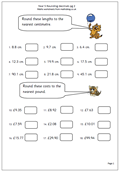The second in our series of rounding worksheets for Year 5 looks at rounding one and two decimal place numbers.

When rounding an amount such as £3.47 to the nearest pound the key digit to look at is the tenths digit. If it is 5 or more, round up. Less than 5, round down.

So £3.44 rounded to the nearest pound is £3.

£3.47 rounded to the nearest pound is £4.

Rounding is very useful when making estimates of answers before using a calculator to make sure that the answer shown on the calculator is sensible.

Rounding decimals p2

## Maths Worksheet: Year 4 Order Decimal Fractions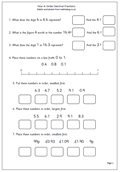It is important that children recognise that the value of a digit depends on its place in the number – otherwise we could only possibly have 9 numbers and zero! So the digit 1 in 120 has a different value than the 1 in 210. It is, of course ten times bigger as it is 100 rather than 10.

By Year 4 children should be applying this idea to decimals. The first column to the right of the decimal point is tenths so the 1 in 2.1 has a value of one tenth.

This maths worksheets looks at both place value and ordering simple decimal fractions. Children probably come across decimal fractions more often with money than any other area and it is a really good way to show place value eg one penny is £0.01, or one hundredth, whilst 10p is £0.10 or one tenth of a pound. (Notice we always put two digits after the decimal when using money, but not at other times.)

Order decimal fractions y4 p1

## Maths Worksheet: Order of Calculating (Bodmas) 3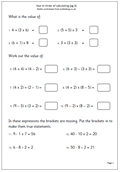This is the third of our worksheets on the order of calculations for Year 6 children, introducing the idea that an expression or row of questions needs to be carried out in a set order so that everyone reaches the same answer.

The first four questions have a single set of brackets, which should be worked out first.

Questions 5 to 10 have two sets of brackets to work out. It is best to encourage a systematic approach, putting in the steps such as:
(4 + 2) x (3 – 1) = 6 x 2 = 12.

Why not just put in the answer? because if an answer is wrong it is much easier to see where the mistake has been made and later at High School students need to show all their working out.

Finally there are a set of expressions which will only be correct if a pair of brackets are entered in the right places.

Order of calculating (Bodmas) pg 3

## Maths Worksheet: Decimal Fractions, hundredths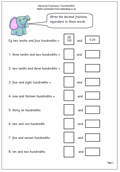Reading decimal fractions can get quite tricky when moving into hundredths and this maths worksheet is a good test of understanding, probably most suited for year 5 children (/10 yrs old).

0.24 can be read as 24 hundredths or 2 tenths and 4 hundredths. As a fraction it would be written as 24/100.

With a number such as five and three hundredths it is important to keep the zero in the tenths so that it is written as 5.03.

Watch out for a common mistake on a question such as twelve hundredths when children write 0.012; the one digit has been placed in the hundredths column when it should be in the tenths with the 2 digit in the hundredths.

Decimal fractions: hundredths (pg 1)

## Maths Worksheet: Rounding Decimals p1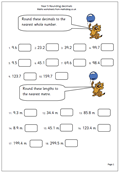Rounding decimals follow the same rules as rounding whole numbers. This first maths worksheet looks at rounding tenths to the nearest whole number. If the tenths are 5 or more round up to the next whole number. If they are less than 5, round down; in other words keep the units the same.

Some children get slightly confused by the term ’round down’ as they think they have to make the units one less (ie 14.3 rounded is 13). Watch out for this mistake.

In real life different rules can apply eg if you have a cupboard 115.4 cm in length it is no use rounding this measurement down to see if it fits in a space. The space may be 115 cm but the cupboard won’t fit!

Rounding decimals (tenths) pg 1

## Y4 Using a Calculator: Take 10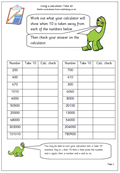Here is the second of our mini series on using a calculator to check the results of mental calculations. In this case 10 has to be n from various numbers , including six digit numbers. Whilst doing these it would be a great idea to practice saying these larger numbers out loud, as many children find this quite difficult and get little chance to practice.

The calculator can be made into a ‘take 10’ machine. With many calculators by keying in the minus sign (-) followed by 10 then the equals sign the answer -10 is shown. Ignore this, don’t clear the answer, and type in another number eg 450 and press the equals sign and the answer will come up (440).

This calculator worksheet can be used by children of different ages, depending on their ability to read and work with large numbers.

Using a calculator: Take 10

Find this worksheet in Year 4 Using and Applying Maths

## Maths Worksheet: Decimal Fractions, tenths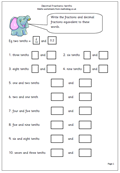Fractions and decimals are two of the sticking points in maths, but here we have a maths worksheet which neatly combines the two, showing the equivalence between fractions and decimal fractions. Many children do not realise the link between a digit written after the decimal point and a fraction i.e.  0.2 is the same as 2/10.

One way to show this is to use the fraction as a division sum. 2/10 can be seen as 2 divided by 10. Do this on a calculator to get 0.2. Also remember, to divide by ten mentally, just move each digit one place to the right, putting in the decimal point if moving from units to tenths.

Decimal fractions: tenths (pg1)

## Coming soon: Bodmas, Decimal Fractions and Rounding DecimalsNext week the third maths worksheet on Bodmas, or the ordering of calculations, will take a closer look at the use of brackets. We will also have pages on decimal fractions. The first will be about rounding decimals and the second on place value and hundredths. Both these are especially suitable for year 5.

The second in our series of using a calculator will look at taking 10 from larger numbers and using the calculator to check mental methods.

## Maths Worksheet: Order of Calculating (Bodmas) 2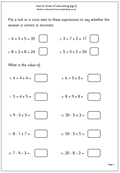The acronym BODMAS
Brackets
Of
Division
Multiplication
Subtraction.
It is a convention to ensure everyone carries out a question such as that below in the same order. Numbers in brackets are worked out first, followed by multiplication and division with addition and subtraction last.

3 + 5 x 2 =

One way to do the question is:       3 + 5 = 8.  8 x 2 = 16.
It can also be done like this:           5 x 2 = 10. 10 + 3 = 13.

If you use a calculator and key in 3 + 5 x 2 you might get 16 or 13, it depends on the type of calculator you use (simple of scientific). So which is ‘correct’?
So with 3 + 5 x 2, the first thing to do is 5 x 2, then add the 3.

To make this easier we often put brackets round parts of the question, to make it clearer, like this:
3 + ( 5 x 2 )
Primary children might not be introduced to the term BODMAS but they should be working in the correct order.

Order of calculating (Bodmas) pg 2

## Year 5 number worksheet: Tests of divisibility for multiples of 3 and 6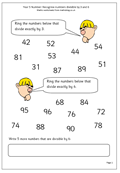This page continues with our series on tests of divisibility.

To find out whether a number is exactly divisible by 3:

Add up the digits of the number.

If the total is divisible by 3 then so is the number.

Eg: 345

3 + 4+ 5 = 12.

12 is divisible by 3 so 345 is also divisible by 3.

To find out if a number is exactly divisible by 6:

Check that it is even.

If it is even, apply the test of divisibility for 3. If the total of the digits is divisible by 3 and the number is even, it is also divisible by 6.

Eg 348

348 is even.

3 + 4+ 8 = 15.

15 is divisible by 3 so 348 is divisible by 6.

Divisible by 3 and 6